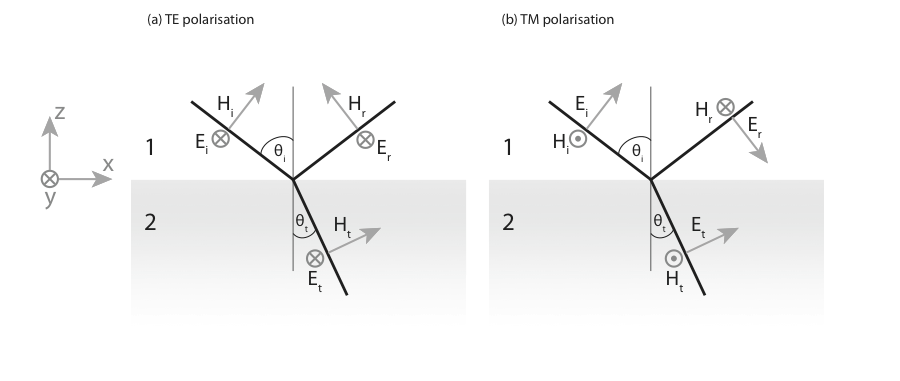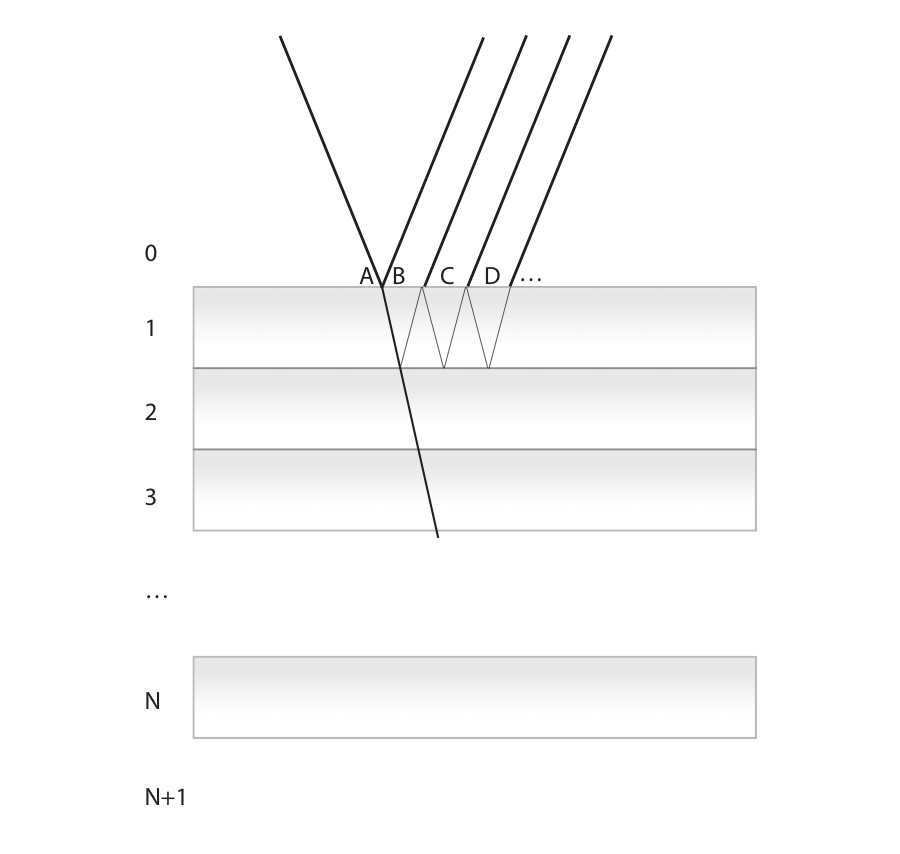# Fresnel formulae

## Single interface

Let us consider the situation depicted below,where we define the Fresnel coefficients as the ratio of the complex amplitude of the electric fields, $$r= E_r/E_i$$ for the reflection coefficient, and $$t= E_t/E_i$$ for the transmission coefficient. The Fresnel coefficients can be obtained by considering the continuity of the tangential component of the $$E$$ and $$H$$ fields at the interface. The continuity of the normal components of $$D$$ and $$B$$ does not yield supplementary conditions and will not be considered. It is worth noting that the continuity of $$H^y$$ is only valid for materials that do not sustain a surface current resulting from the addition of external charges.

### TE-polarised light

For TE-polarised light, the continuity of $$E^y$$ reads, $E_i + E_r = E_t$ which yields, $1+r =t.$ The continuity of $$H^x$$ can be written as, $H_i\cos\theta_i - H_r\cos\theta_i = H_t\cos\theta_t$ It is convenient to express the angles in terms of the normal component of the $$k$$-vectors, $k_z = n k_0 \cos\theta.$ for the incident, reflected, and refracted fields, so that $$\cos\theta_t / \cos\theta_i=\dfrac{k_{z2}n_1}{k_{z1}n_2}$$. The complex magnitude of the magnetic and electric fields are linked in each medium by the optical impedance. Its expression can be obtained from the induction law in a homogeneous medium, $\nabla\times\mathbf{E} = -\partial_t \mathbf{B}$ expressed in Fourier space\footnote{assuming here a homogeneous plane wave} as, $\mathbf{k}\times \mathbf{E} = i\omega \mathbf{B}$ The ratio $$E/H$$ is the impedance, written as, $\frac{E}{H}= c \mu \mu_0 / n= \sqrt{\frac{\mu\mu_0}{\varepsilon\varepsilon_0}}=Z\cdot Z_0.$ We can define the admittance $$Y$$ as the inverse impedance, $$Y=\dfrac{1}{Z\cdot Z_0}$$ The continuity of $$H^y$$ can be expressed as, $Y_1 (1 - r) = Y_2 \frac{n_1k_{z2}}{n_2k_{z1}}t.$ After rearranging, we obtain, $1 - r = \frac{\mu_1k_{z2}}{\mu_2k_{z1}}t.$ We can summarize the two continuity relations in the following system,

\left\{ \begin{aligned} 1 + r &= t\ 1 - r &= \frac{\mu_1 k_{z2}}{\mu_2 k_{z1}}t \end{aligned}\right. Solving for $$r$$ and $$t$$ yields the result, $t_{12}^s=\frac{2\mu_2 k_{z1}}{\mu_2 k_{z1}+\mu_1k_{z2}},\qquad r_{12}^s=\frac{\mu_2 k_{z1}-\mu_1k_{z2}}{\mu_2 k_{z1}+\mu_1k_{z2}}.$

### TM-polarised light

For TM-polarised light, it is generally easier to consider the Fresnel coefficients for the magnetic field, denoted $$\rho$$ and $$\tau$$. The continuity of $$H^y$$ reads, $1 - \rho = \tau$ The continuity of $$E^y$$ can be written, $\frac{k_{z1}}{\varepsilon_1} + \rho \frac{k_{z1}}{\varepsilon_1} = \tau \frac{k_{z2}}{\varepsilon_2}$

We can summarize the two continuity relations in the following system,

\left\{ \begin{aligned} 1 - rho &=\tau\ (1 + \rho) \frac{k_{z1}}{\varepsilon_1}&=\frac{k_{z2}}{\varepsilon_2}\tau \end{aligned}\right. Solving for $$\rho$$ and $$\tau$$ yields the result, $\tau_{12}^p=\frac{2\varepsilon_2 k_{z1}}{\varepsilon_2 k_{z1}+\varepsilon_1k_{z2}},\qquad \rho_{12}^p=\frac{\varepsilon_2 k_{z1}-\varepsilon_1k_{z2}}{\varepsilon_2 k_{z1}+\varepsilon_1k_{z2}}.$

To summarize, for a single interface from 1 to 2 with normal along the $$z$$ direction, the Fresnel coefficients read, \begin{aligned} \rho_{12}^p&=\frac{\varepsilon_2 k_{z1}-\varepsilon_1k_{z2}}{\varepsilon_2 k_{z1}+\varepsilon_1k_{z2}},&{}& r_{12}^s&=\frac{\mu_2 k_{z1}-\mu_1k_{z2}}{\mu_2 k_{z1}+\mu_1k_{z2}}\ \tau_{12}^p&=\frac{2\varepsilon_2 k_{z1}}{\varepsilon_2 k_{z1}+\varepsilon_1k_{z2}},&{}& t_{12}^s&=\frac{2\mu_2 k_{z1}}{\mu_2 k_{z1}+\mu_1k_{z2}}. \end{aligned}

Note that, $r_{ij}=-r_{ji}.$ To verify the conservation of energy, one must consider the irradiance defined in terms of the electric field as $$I=\frac{1}{2}Y|E|^2$$, yielding, $R=\frac{I_r}{I_i}=|r|^2, \qquad T=\frac{I_t}{I_i}=\frac{Y_1}{Y_2}|t|^2.$ and we can verify that, indeed, $$R+T=1$$ for a single interface.

## Reflectivity of a layer

From the viewpoint of ray optics, a thin layer will support an infinite number of internal reflections (absorption and irregularities will however reduce the intensity in a physical situation). The infinite series of reflected orders can be expressed in the form a geometric sum, leading to a closed form formula as shown below.An incident plane wave with amplitude $$A$$ impinges on the first interface. It can be reflected, $$B=r_{01}A$$, or transmitted. The total response of the slab can be obtained by following each order of reflection inside the slab (C',D', \dots).

Upon transmission at the first interface, the wave amplitude is $$t_{01}A$$. Application of Fermat's principle yields a phase change $$\Delta \phi= k_{z1}d$$ when the wave hits the second interface. The reflection coefficient at this interface is $$r_{12}$$. The partial wave reflected from this path, noted C', is therefore $$C=t_{10}t_{01}r_{12}\exp(2i k_{z1}d)A$$.

Similarly, in D', $D=t_{10}t_{01}r_{10}r_{12}^2\exp(4i k_{z1}d)A$ And, for the $${j^\text{th}}$$ partial wave, $t_{10}t_{01}r_{12}^{j}r_{10}^{{j-1}}\exp(2i {j}k_{z1}d)A$ The wave reflected by the slab is the sum of these contributions, $r_{\text{slab}}A=B+C+D+\dots=\left[r_{01}+t_{10}t_{01}r_{12}\sum_{j=0}^{\infty}r_{12}^jr_{10}^{j}\exp(2ji k_{z1} d)\right]A$ For clarity, I write $$\beta=r_{12}r_{10}\exp(2i k_{z1}d)$$. The summation of all partial waves is thereby expressed as a geometrical sum, $r_{\text{slab}}=r_{01} + \left(t_{10}t_{01}r_{12}\exp(2i k_{z1}d)\right)\sum_{j=0}^{\infty}\beta^j$ Recalling that the sum of a geometric series of common ratio $$q$$ is $$\frac{1}{1-q}$$, we can write, $r_{\text{slab}}=r_{01} + \frac{t_{10}t_{01}r_{12}\exp(2i k_{z1}d)}{1-r_{12}r_{10}\exp(2i k_{z1}d)}$

We note that for either polarisation we have the following identity, $t^s_{ij}t^s_{ji} = (1-r^s_{ij})(1+r^s_{ij})= 1-(r^s_{ij})^2 ,\qquad t^p_{ij}t^p_{ji} =\frac{Y_i}{Y_j}(1-r^p_{ij})\frac{Y_j}{Y_i}(1+r^p_{ij}) = 1-(r^p_{ij})^2$ Using this equation and the substitution $$r_{10} = -r_{01}$$ we finally obtain, $r_{\text{slab}}=\frac{r_{01}+r_{12}\exp(2i k_{z1}d)}{1+r_{01}r_{12}\exp(2i k_{z1}d)}.$

For the transmission, one obtains,

$t_{\text{slab}}=\frac{t_{01}t_{12}\exp(i k_{z1}d)}{1+r_{01}r_{12}\exp(2i k_{z1}d)}.$

## Reflectivity of a multilayer structure

When N layers are stacked together, the reflection coefficient of the structure can be found by applying recursively the preceding formula for a single layer. This amounts to considering one of the reflection coefficients to be the effective reflection accounting for all the layers behind. Let us consider explicitly the case of a 3 layers system. The procedure is as follows,

• Compute and store for later use all single-interface coefficients, namely $$r_{01}$$, $$r_{12}$$, $$r_{23}$$, $$r_{34}$$
• Combine the previous coefficients to compute the reflectivity of the last layer, i.e. $$r_{24} = \frac{r_{23}+r_{34}\exp(2i k_{z3}d_3)}{1+r_{23}r_{34}\exp(2i k_{z3}d_3)}$$. This value can be stored in a temporary variable $$r_{\text{tmp}}$$
• Go up, combining one interface at a time, $$r_{\text{tmp}}=r_{14} = \frac{r_{12}+r_{24}\exp(2i k_{z2}d_2)}{1+r_{12}r_{24}\exp(2i k_{z2}d_2)}$$
• the combined reflectivity of the multilayer is obtained when one has reached the top interface, $$r_{\text{tmp}} = r_{04} = \frac{r_{01}+r_{14}\exp(2i k_{z1}d_1)}{1+r_{01}r_{14}\exp(2i k_{z1}d_1)}$$